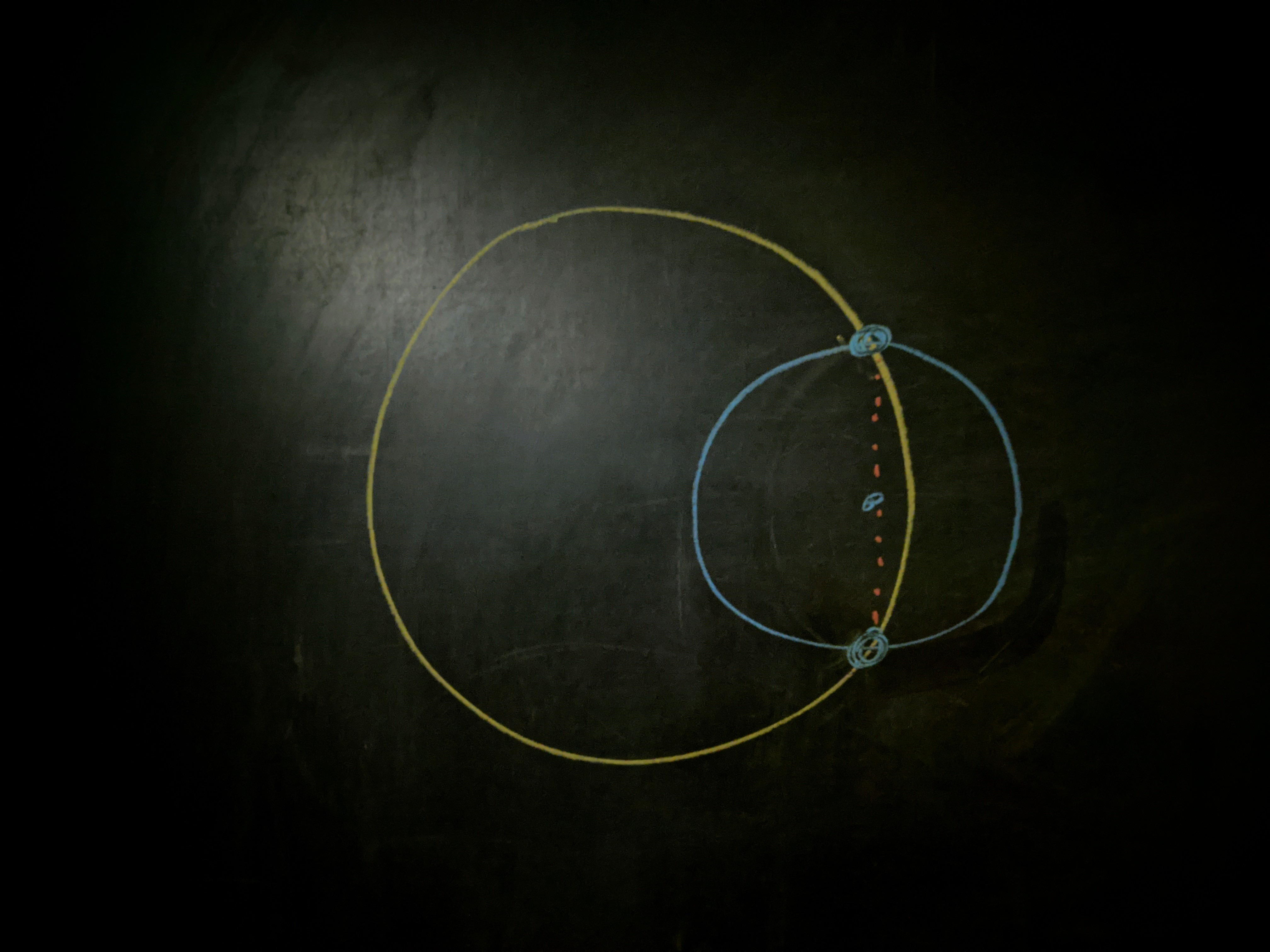Question: Trapped in his house under Stage $4$ lockdown, Zach sits at the kitchen table with a half full glass and a cardboard disc, upon which is written the password he uses to edit the Riddler. Suddenly, he hears someone talking about cranberry sauce in the other room. He just can’t get enough of that cranberry sauce! When he gets up, he places the disc on the rim of the glass such that the disc’s center is at a random location on the inside of the rim. What is the probability that the cardboard disc falls into the half full glass of water, dissolving the password and forcing the Riddler into a $2$-week hiatus, because that is the only explanation for why Zach would abandon us like this?

## Solution

The disc — as with all objects — will tip if its center of mass extends beyond the edge of what it’s resting on. The first part is simple for the disc; due to its radial symmetry, its center of mass is at its center. The second part isn’t as clear.

### The edge(s) of the glass

With a straight object over a straight edge — like a book off a desk — the edge is obvious.

On the rim there are two answers: the disc can fall off the glass or into it.

To fall off the glass, the center of the disc has to extend beyond the rim. Beyond the rim, gravity will pull the center of mass down with no force to tilt it back up.

### When do we fall in?

Falling in is another story. The center of the disc can extend over the inside of the glass — being pulled down by gravity — while the glass exerts a force to tilt the disc back up. This is because the glass exerts force on the disc at the points of contact.

When the center of mass first breaches the inner rim, the points of contact are further in than the center of mass. But as we continue to push the disc, the angle between the points of contact and the center of mass grows until the three points are lined up. This is the furthest inward we can place the disc because the downward pull of gravity an the upward force of the glass are balanced, and there’s no imbalance of tilting forces. If we were to push it any further then the points of contact wouldn’t be able to provide a tilting force to oppose gravity and the disc would fall in.Small disc balanced as far in as possible while maintaining a stable perch.

So, if the center of mass is anywhere from the outer rim of the glass to the radius where the center of mass becomes colinear with the points of contact, the disc will stay up. As we’ve shown, this happens when the chord connecting the points of contact is equal to the diameter of the smaller disc.

Using the Pythagorean theorem, this radius is

$r_\text{unstable} = \sqrt{R^2 - r^2}.$

### Will we fall in?

Assuming that we place the center of the disc at a random point inside the rim, the probability that the disc stays up is equal to the area of the annular region between $r_\text{unstable}$ and $R:$

\begin{align} P_\text{stable} &= \dfrac{A_\text{stable}}{A_\text{total}} \\ &= \dfrac{A_\text{total} - A_\text{unstable}}{A_\text{total}} \\ &= \dfrac{\pi R^2 - \pi {r_\text{unstable}}^2}{\pi R^2} \\ &= \left(\dfrac{r}{R}\right)^2 \end{align}

For the disc in question, this probability is $\boxed{P_\text{stable} = 1/2^2 = 1/4}.$